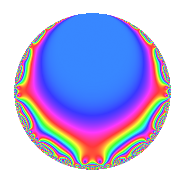Properties

 Label 8034.2.a.mLevel 8034 Weight 2 Character orbit 8034.a Self dual Yes Analytic conductor 64.152 Analytic rank 1 Dimension 2 CM No Inner twists 1

Related objects

Newspace parameters

 Level: $$N$$ = $$8034 = 2 \cdot 3 \cdot 13 \cdot 103$$ Weight: $$k$$ = $$2$$ Character orbit: $$[\chi]$$ = 8034.a (trivial)

Newform invariants

 Self dual: Yes Analytic conductor: $$64.1518129839$$ Analytic rank: $$1$$ Dimension: $$2$$ Coefficient field: $$\Q(\sqrt{65})$$ Coefficient ring: $$\Z[a_1, \ldots, a_{17}]$$ Coefficient ring index: $$1$$ Fricke sign: $$1$$ Sato-Tate group: $\mathrm{SU}(2)$

$q$-expansion

Coefficients of the $$q$$-expansion are expressed in terms of $$\beta = \frac{1}{2}(1 + \sqrt{65})$$. We also show the integral $$q$$-expansion of the trace form.

 $$f(q)$$ $$=$$ $$q$$ $$- q^{2}$$ $$- q^{3}$$ $$+ q^{4}$$ $$+ q^{6}$$ $$+ q^{7}$$ $$- q^{8}$$ $$+ q^{9}$$ $$+O(q^{10})$$ $$q$$ $$- q^{2}$$ $$- q^{3}$$ $$+ q^{4}$$ $$+ q^{6}$$ $$+ q^{7}$$ $$- q^{8}$$ $$+ q^{9}$$ $$+ 3 q^{11}$$ $$- q^{12}$$ $$+ q^{13}$$ $$- q^{14}$$ $$+ q^{16}$$ $$+ ( 2 - \beta ) q^{17}$$ $$- q^{18}$$ $$- q^{21}$$ $$-3 q^{22}$$ $$+ ( -3 + \beta ) q^{23}$$ $$+ q^{24}$$ $$-5 q^{25}$$ $$- q^{26}$$ $$- q^{27}$$ $$+ q^{28}$$ $$+ 4 q^{31}$$ $$- q^{32}$$ $$-3 q^{33}$$ $$+ ( -2 + \beta ) q^{34}$$ $$+ q^{36}$$ $$+ ( -3 - \beta ) q^{37}$$ $$- q^{39}$$ $$+ ( -1 + \beta ) q^{41}$$ $$+ q^{42}$$ $$+ ( -1 + \beta ) q^{43}$$ $$+ 3 q^{44}$$ $$+ ( 3 - \beta ) q^{46}$$ $$+ ( -5 + \beta ) q^{47}$$ $$- q^{48}$$ $$-6 q^{49}$$ $$+ 5 q^{50}$$ $$+ ( -2 + \beta ) q^{51}$$ $$+ q^{52}$$ $$+ ( 2 - \beta ) q^{53}$$ $$+ q^{54}$$ $$- q^{56}$$ $$-2 \beta q^{59}$$ $$+ ( -7 + \beta ) q^{61}$$ $$-4 q^{62}$$ $$+ q^{63}$$ $$+ q^{64}$$ $$+ 3 q^{66}$$ $$+ ( -8 - \beta ) q^{67}$$ $$+ ( 2 - \beta ) q^{68}$$ $$+ ( 3 - \beta ) q^{69}$$ $$+ ( -8 + 2 \beta ) q^{71}$$ $$- q^{72}$$ $$+ ( -6 + 3 \beta ) q^{73}$$ $$+ ( 3 + \beta ) q^{74}$$ $$+ 5 q^{75}$$ $$+ 3 q^{77}$$ $$+ q^{78}$$ $$-2 \beta q^{79}$$ $$+ q^{81}$$ $$+ ( 1 - \beta ) q^{82}$$ $$+ 2 \beta q^{83}$$ $$- q^{84}$$ $$+ ( 1 - \beta ) q^{86}$$ $$-3 q^{88}$$ $$+ ( -4 - 2 \beta ) q^{89}$$ $$+ q^{91}$$ $$+ ( -3 + \beta ) q^{92}$$ $$-4 q^{93}$$ $$+ ( 5 - \beta ) q^{94}$$ $$+ q^{96}$$ $$-4 \beta q^{97}$$ $$+ 6 q^{98}$$ $$+ 3 q^{99}$$ $$+O(q^{100})$$ $$\operatorname{Tr}(f)(q)$$ $$=$$ $$2q$$ $$\mathstrut -\mathstrut 2q^{2}$$ $$\mathstrut -\mathstrut 2q^{3}$$ $$\mathstrut +\mathstrut 2q^{4}$$ $$\mathstrut +\mathstrut 2q^{6}$$ $$\mathstrut +\mathstrut 2q^{7}$$ $$\mathstrut -\mathstrut 2q^{8}$$ $$\mathstrut +\mathstrut 2q^{9}$$ $$\mathstrut +\mathstrut O(q^{10})$$ $$2q$$ $$\mathstrut -\mathstrut 2q^{2}$$ $$\mathstrut -\mathstrut 2q^{3}$$ $$\mathstrut +\mathstrut 2q^{4}$$ $$\mathstrut +\mathstrut 2q^{6}$$ $$\mathstrut +\mathstrut 2q^{7}$$ $$\mathstrut -\mathstrut 2q^{8}$$ $$\mathstrut +\mathstrut 2q^{9}$$ $$\mathstrut +\mathstrut 6q^{11}$$ $$\mathstrut -\mathstrut 2q^{12}$$ $$\mathstrut +\mathstrut 2q^{13}$$ $$\mathstrut -\mathstrut 2q^{14}$$ $$\mathstrut +\mathstrut 2q^{16}$$ $$\mathstrut +\mathstrut 3q^{17}$$ $$\mathstrut -\mathstrut 2q^{18}$$ $$\mathstrut -\mathstrut 2q^{21}$$ $$\mathstrut -\mathstrut 6q^{22}$$ $$\mathstrut -\mathstrut 5q^{23}$$ $$\mathstrut +\mathstrut 2q^{24}$$ $$\mathstrut -\mathstrut 10q^{25}$$ $$\mathstrut -\mathstrut 2q^{26}$$ $$\mathstrut -\mathstrut 2q^{27}$$ $$\mathstrut +\mathstrut 2q^{28}$$ $$\mathstrut +\mathstrut 8q^{31}$$ $$\mathstrut -\mathstrut 2q^{32}$$ $$\mathstrut -\mathstrut 6q^{33}$$ $$\mathstrut -\mathstrut 3q^{34}$$ $$\mathstrut +\mathstrut 2q^{36}$$ $$\mathstrut -\mathstrut 7q^{37}$$ $$\mathstrut -\mathstrut 2q^{39}$$ $$\mathstrut -\mathstrut q^{41}$$ $$\mathstrut +\mathstrut 2q^{42}$$ $$\mathstrut -\mathstrut q^{43}$$ $$\mathstrut +\mathstrut 6q^{44}$$ $$\mathstrut +\mathstrut 5q^{46}$$ $$\mathstrut -\mathstrut 9q^{47}$$ $$\mathstrut -\mathstrut 2q^{48}$$ $$\mathstrut -\mathstrut 12q^{49}$$ $$\mathstrut +\mathstrut 10q^{50}$$ $$\mathstrut -\mathstrut 3q^{51}$$ $$\mathstrut +\mathstrut 2q^{52}$$ $$\mathstrut +\mathstrut 3q^{53}$$ $$\mathstrut +\mathstrut 2q^{54}$$ $$\mathstrut -\mathstrut 2q^{56}$$ $$\mathstrut -\mathstrut 2q^{59}$$ $$\mathstrut -\mathstrut 13q^{61}$$ $$\mathstrut -\mathstrut 8q^{62}$$ $$\mathstrut +\mathstrut 2q^{63}$$ $$\mathstrut +\mathstrut 2q^{64}$$ $$\mathstrut +\mathstrut 6q^{66}$$ $$\mathstrut -\mathstrut 17q^{67}$$ $$\mathstrut +\mathstrut 3q^{68}$$ $$\mathstrut +\mathstrut 5q^{69}$$ $$\mathstrut -\mathstrut 14q^{71}$$ $$\mathstrut -\mathstrut 2q^{72}$$ $$\mathstrut -\mathstrut 9q^{73}$$ $$\mathstrut +\mathstrut 7q^{74}$$ $$\mathstrut +\mathstrut 10q^{75}$$ $$\mathstrut +\mathstrut 6q^{77}$$ $$\mathstrut +\mathstrut 2q^{78}$$ $$\mathstrut -\mathstrut 2q^{79}$$ $$\mathstrut +\mathstrut 2q^{81}$$ $$\mathstrut +\mathstrut q^{82}$$ $$\mathstrut +\mathstrut 2q^{83}$$ $$\mathstrut -\mathstrut 2q^{84}$$ $$\mathstrut +\mathstrut q^{86}$$ $$\mathstrut -\mathstrut 6q^{88}$$ $$\mathstrut -\mathstrut 10q^{89}$$ $$\mathstrut +\mathstrut 2q^{91}$$ $$\mathstrut -\mathstrut 5q^{92}$$ $$\mathstrut -\mathstrut 8q^{93}$$ $$\mathstrut +\mathstrut 9q^{94}$$ $$\mathstrut +\mathstrut 2q^{96}$$ $$\mathstrut -\mathstrut 4q^{97}$$ $$\mathstrut +\mathstrut 12q^{98}$$ $$\mathstrut +\mathstrut 6q^{99}$$ $$\mathstrut +\mathstrut O(q^{100})$$

Embeddings

For each embedding $$\iota_m$$ of the coefficient field, the values $$\iota_m(a_n)$$ are shown below.

For more information on an embedded modular form you can click on its label.

Label $$\iota_m(\nu)$$ $$a_{2}$$ $$a_{3}$$ $$a_{4}$$ $$a_{5}$$ $$a_{6}$$ $$a_{7}$$ $$a_{8}$$ $$a_{9}$$ $$a_{10}$$
1.1
 4.53113 −3.53113
−1.00000 −1.00000 1.00000 0 1.00000 1.00000 −1.00000 1.00000 0
1.2 −1.00000 −1.00000 1.00000 0 1.00000 1.00000 −1.00000 1.00000 0
 $$n$$: e.g. 2-40 or 990-1000 Significant digits: Format: Complex embeddings Normalized embeddings Satake parameters Satake angles

Inner twists

This newform does not admit any (nontrivial) inner twists.

Atkin-Lehner signs

$$p$$ Sign
$$2$$ $$1$$
$$3$$ $$1$$
$$13$$ $$-1$$
$$103$$ $$-1$$

Hecke kernels

This newform can be constructed as the intersection of the kernels of the following linear operators acting on $$S_{2}^{\mathrm{new}}(\Gamma_0(8034))$$:

 $$T_{5}$$ $$T_{7}$$ $$\mathstrut -\mathstrut 1$$